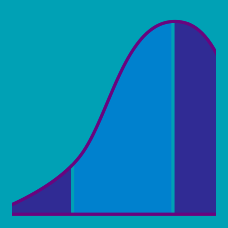Probability

# Continuous Random Variables - Joint Probability Distribution

Let $X$ and $Y$ have the joint probability density function: $f(x,y)=\frac{1}{49}xy$ for $0\leq x\leq2$ and $0\leq y\leq7.$ Find the expected value of $3X.$

Let $X$ and $Y$ have the joint probability density function: $f(x,y)=k(x+y)$ for $0\leq x\leq10$ and $0\leq y\leq1,$ where $k$ is a constant. Find the marginal probability $P(1\leq X\leq2).$

Let $X$ and $Y$ have the joint probability density function: $f(x,y)=k$ for $0\leq x\leq5$ and $0\leq y\leq8,$ where $k$ is a constant. Find the covariance between $X$ and $Y.$

Let $X$ and $Y$ have the joint probability density function: $f(x,y)=kxy$ for $0\leq x\leq4$ and $0\leq y\leq3,$ where $k$ is a constant. Find $P(X < Y).$

Which of the following CANNOT be the joint probability density function of two continuous random variables $X$ and $Y?$

(A): $f(x,y)=1$ for $0\leq x,y\leq1.$

(B): $f(x,y)=x+y$ for $0\leq x,y\leq1.$

(C): $f(x,y)=xy$ for $0\leq x,y\leq1.$

(D): $f(x,y)=e^{x+y}$ for $0\leq x,y\leq\ln2.$

×